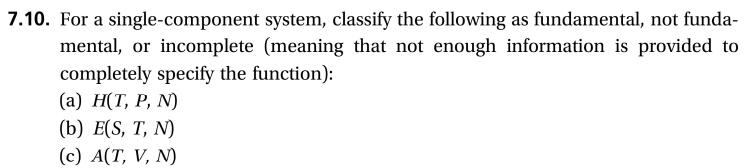# 7.10. For a single-component system, classify the following as fundamental, not funda-incomplete (meaning that not enough information is provided tomental, orcompletely specify the function):(а) Н(Т, Р, N)(b) E(S, T, N)(c) A(T, V, N)

Question
1 views

This problem is (7.10) from a book  "Thermodynamics and Statistical Mechanics An Integrated Approach by M. Scott Shell"help_outlineImage Transcriptionclose7.10. For a single-component system, classify the following as fundamental, not funda- incomplete (meaning that not enough information is provided to mental, or completely specify the function): (а) Н(Т, Р, N) (b) E(S, T, N) (c) A(T, V, N) fullscreen
check_circle

Step 1

Fundamental functions are those functions which are formed with the help of intensive variables (independent of mass).

And derived functions are those functions which are formed with the help of fundamental functions.

Step 2

(a). Enthalpy is the sum of internal energy and work (flow work)

Where N= 1 (for a single-component system)

T is tempreture

P is pressure

Function is calculated as follows with the help pf partial derivative

Step 3

From the fundamental equation

Enthalpy is function of entropy (S) and pressure (P) not temperature (T) and pressure

dH is given as follows:

Where S is entropy

&nbs...

### Want to see the full answer?

See Solution

#### Want to see this answer and more?

Solutions are written by subject experts who are available 24/7. Questions are typically answered within 1 hour.*

See Solution
*Response times may vary by subject and question.
Tagged in

### Thermodynamics